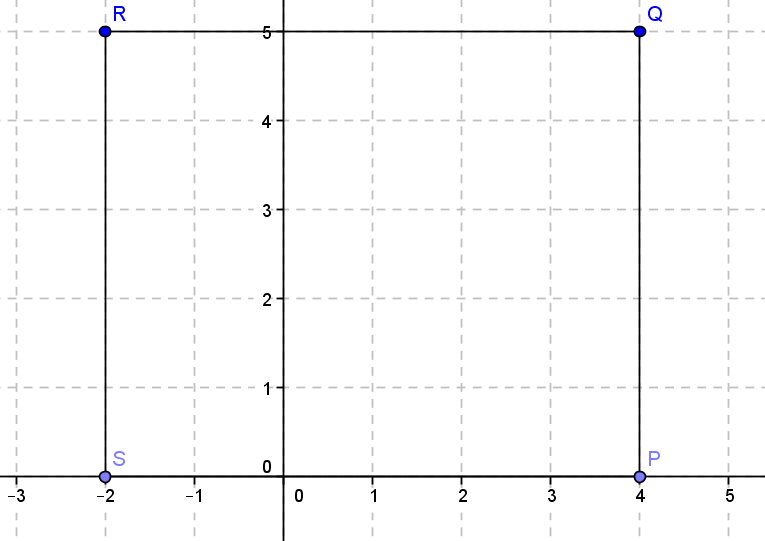# Mock Exam Paper D P2 (Project Maths) Foundation Level Marking Scheme

Leaving Cert Examination 2014

Mathematics

(Projects Maths – Phase 3)

Paper II

Foundation Level

Time hours

300 marks

Model Solutions – Paper 1

Note the model solutions for each question are not intended to be exhaustive – there may be other correct solutions.

Instructions

There are two sections in this examination paper:

Section A Concept and skills 200 marks 8 questions

Section B Contexts and Applications 100 marks 2 questions

Answer all eight questions as follows:

Questions 1 to 7 and

either Question 8A or Question 8B

In section B, answer Question 9 and Question 10.

Write your answers in the spaces provided in this booklet. There is space for extra work at the back of the booklet. You may also ask the superintendent for more paper. Label any extra paper clearly with the question number and part.

The superintendent will give you a copy of the booklet of Formulae and Tables. You must return is at the end of the examination. You are not allowed to bring your won copy into the examination.

Marks will be lost if all necessary work is not clearly shown.

Answers should include the appropriate units of measurement, where relevant.

Answers should be given in simplest form, where relevant.

Write the mark and model of your calculator(s) here:

Section A Concept and skills 150 marks

Question 1 25 marks

A plastics firm is asked to make a cube of side 5cm container, as shown.

In order to make this the company must first make a net of the object. Draw a circle around any net below which of the following could be folded to form the cube.

(b) Find the volume of one cube

Vol =

(c) If the cubes are stacked to form a larger cube with side 15cm. How many cubes were used?

9 cubes

Question 2 25 marks(i) Write down the coordinates of the point Q

(ii) Plot the points , , and on the coordinate plane above.

(iii) Join the points P,Q,R and S. What shape have your draw. Rectangel

How many axis of symmetry does this shape have? 2

(v) Find the slope of th...